Back to Roger's Main Page

## Roger's Online Equation Editor

This page produces high quality typeset images of equations from LaTeX equations. These images can be used on web pages or in other documents.

This page is a PHP implementation of the tex2im bash script created by Andreas Reigber. If you have questions or problems with this page please email me. The page takes a few seconds to generate an image.

The "transparent background" option is particularly useful for the web. All file formats except JPG support a transparent background. In general, you will get poor results if you use both "transparent background" and "anti aliasing" at the same time. The note here explains why.

You do NOT need to include either the $...$ tags (for math environment) or \begin{equation} ... \end{equation} tags (for equation environment) in your LaTeX string. Because the the latex code you enter will be placed inside a \begin{eqnarray*}...\end{eqnarray*} environment. If you don't know what I'm talking about don't worry. Just use the examples below.

Note about the "|" character: To use the "|" character in an equation, use the command "\vbar", otherwise using mutiple instances of "|" can cause a server error.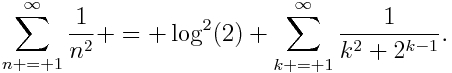Clear Entry

 Latex Equation String \sum_{n = 1}^\infty\frac{1}{n^2} = \log^2(2)+\sum_{k = 1}^\infty\frac{1}{k^2 2^{k-1}}. Output Format: png jpg tif gif Background Color: white black red yellow blue green magenta cyan Text Color: white black red yellow blue green magenta cyan Resolution: Transparent Backgound Anti Alias Text

## Writing Equations in LaTeX

Sample Equations and their LaTex String
Subscripts and Superscripts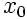x_{0}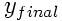y_{final}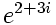e^{2+3i}
Fractions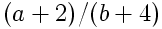(a+2)/(b+4)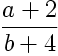\frac{a+2}{b+4}
Integrals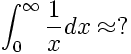\int_0^{\infty} \frac{1}{x} dx \approx ?
Misc.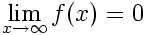\lim_{x\to\infty}f(x)=0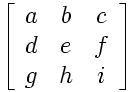$\left[ \begin{array}{ccc} a & b & c \\\ d & e & f \\\ g & h & i \end{array} \right]$
Note: An extra slash is needed (beyond LaTex's 2) at the end of each line.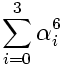\sum_{i=0}^3 \alpha_i^6
Note: Greek letters are generated by typing \alpha \beta \gamma \delta etc.

Note: Using "transparent background" and "anti-alias" at the same time can produce poor quality image edges. The anti-aliasing is done with a color halfway between text color and the selected background color. However, only the selected background color is made transparent (leaving the halfway color behind on the edges). The image will look ok if the selected background color is similar to the color the transparent equation will be put on top of.

To see this effect, enter an equation (size 300 or bigger so you can see the edges clearly) and select both the "transparent background" and "anti aliasing" option. Now view it using a variety of background colors. You can see how the edges of the image change colors. The "transparent background" feature does not seem to work if the background color is selected to green.